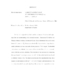## Charge Form Factor of the Neutron Through d vector(e vector, e'n) at Q(2) = 1.0(GeV/c)2##### Files
dissertation.pdf(3.4 MB)
Elastic electromagnetic form factors of the nucleon are of fundamental importance for our understanding of its internal structure. Experiment E93-026 at the Thomas Jefferson National Accelerator Facility (JLab) determined the electric form factor of the neutron, $G_E^n$, through quasielastic ${\vec{d}(\vec{e},e'n)p}$ scattering using a longitudinally polarized electron beam and a frozen polarized $^{15}ND_3$ target. The knocked out neutrons were detected in a segmented plastic scintillator detector in coincidence with the scattered electrons, which were tracked in High Momentum Spectrometer of the Hall C. The form factor was extracted by comparing the experimental beam--target asymmetry with full theoretical calculations based on different values of $G_E^n$. The dissertation discusses the experimental setup, data acquisition and analysis for the $Q^2=1.0~(\mathrm{GeV}/c)^2$ point, and implications of the experimental results for our understanding of the nucleon electromagnetic structure.